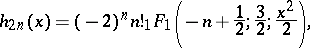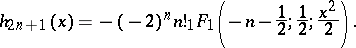# Hermite function

(diff) ← Older revision | Latest revision (diff) | Newer revision → (diff)

A solution of the Hermite equation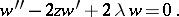The Hermite functions have the form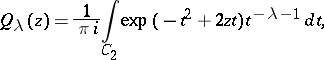where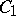is the contour in the complex-plane consisting of the rays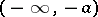and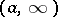and the semi-circle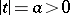,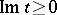, and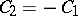. The half-sum of these solutions,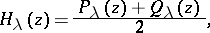for an integer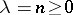, is equal to the Hermite polynomial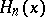(cf. Hermite polynomials). The name Hermite equation is also used for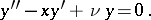Whenis an integer, this equation has the fundamental system of solutions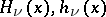, where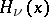are the Hermite polynomials and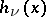are the Hermite functions of the second kind, which can be expressed in terms of the confluent hypergeometric function: